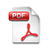On Estimating the Size and Confidence of a Statistical Audit
Working Paper No.:  54
Date Published:  2008-11-30

#### Author(s):

Javed A. Aslam, Northeastern University

Raluca A. Popa, Massachusetts Institute of Technology

Ronald L. Rivest, Massachusetts Institute of Technology

#### Abstract:

We consider the problem of statistical sampling for auditing elections, and we develop a remarkably simple and easily-calculated upper bound for the sample size necessary for determining with probability at least c whether a given set of n objects contains b or more “bad” objects. While the size of the optimal sample drawn without replacement can be determined with a computer program, our goal is to derive a highly accurate and simple formula that can be used by election officials equipped with only a simple calculator. We actually develop several formulae, but the one we recommend for use in practice is: U3(n, b, c) = ln − (b − 1) 2  ·  1 − (1 − c) 1/bm = ln − (b − 1) 2  ·  1 − exp(ln(1 − c)/b) m As a practical matter, this formula is essentially exact: we prove that it is never too small, and empirical testing for many representative values of n ≤ 10, 000, and b ≤ n/2, and c ≤ 0.99 never finds it more than one too large. Theoretically, we show that for all n and b this formula never exceeds the optimal sample size by more than 3 for c ≤ 0.9975, and by more than (− ln(1−c))/2 for general c.

#### AttachmentOn Estimating the Size and Confidence of a Statistical Audit  (Size: 245 KB)